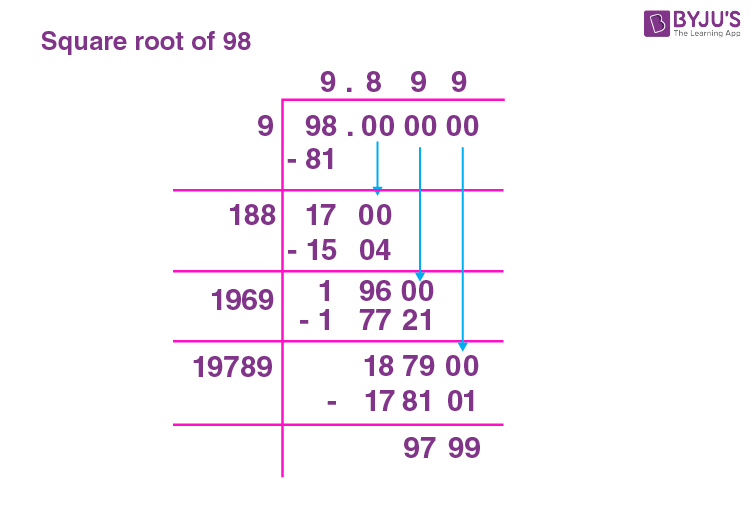# Square Root of 98

The square root of 98 is irrational. For the given number “n” to have a square root, it should have a factor “f” such that f × f = n. In other words, when a number is multiplied by itself, the product is called the perfect square. The number that is multiplied is called the square root. Consider the number 9 × 9 = 81 and 10 × 10 = 100. 98 lies between 81 and 100. So the square root should also lie between 9 and 10. Therefore, 98 is not a perfect square number and its root is also not perfect, as it does not have a number, which, when multiplied by itself gives 98. Therefore, √98 is an irrational number. You can refer to irrational numbers.

Note the Following:

• The Square root of 98 = √98 where ‘√’ is the radical, and 98 is the radicand.
• Exponential Form of Square root of 98 = 981/2
• Solution for √98 ≈ 9.8
• The square root of 98 is Irrational = True

## What is the Square root of 98?

The square root of 98 is 9.8, as the square root of 98 is irrational.

 √98 = 7√2 = 9.899

## How to Find the Square root of 98?

There are three methods to find the Square root of 98

• Prime Factorisation method
• Long Division method
• Repeated Subtraction method

### Square root of 98 by Prime Factorisation Method

In the prime factorisation method, identifying the square root of a number can be done by dividing the number by prime factors. Group 2 same prime factors and the product of the grouped prime factors form the square root. Let us divide the number 98 by the prime factors, starting with the smallest, i.e., 2.

The given number, 98 will be expressed as;

 2 98 7 49 7 7 × 1

98 = 2 × 7 × 7

Grouping into 2 with same divisors:

Group 1 = 7 × 7, considering only 7

Another divisor 2 is left out as it does not have a pair.

Therefore the square root of 98 = 7× √2 = 7√2.

### Square root of 98 by Long Division Method

To understand the long division method, follow the below mentioned detailed steps;

Step 1:  Grouping the given number into pairs.

Step 2: Consider the first number, and use the divisor such that the product is less than or equal to the first number. The divisors should be:

1 × 1 = 1

2 × 2 = 4

3 × 3 = 9

Step 3: Continue the division, by bringing the next pair

Step 4: The first divisor in the next division to be  (Divisor of first division + quotient of first division) such that:

d1 × 1 =

d2 × 2 =

d3 x 3 =

And so on.

Step 5: If the division is complete with the remainder as zero, then the quotient will be the square root. Else the number is not a perfect square number.

Applying the above steps to 98, the squares are:

8 × 8 = 64

9 × 9 = 81

10 × 10 = 100No further division is possible and the remainder is non-zero. Hence 98 does not have a perfect square. Therefore 98 is an irrational number which is 7√2.

### Square root of 98 by Repeated Subtraction Method.

To find the solution using repeated subtraction, the given number is subtracted repeated by the odd numbers starting from 1. If the difference at any point of subtraction becomes zero, then the given number is a perfect square, else it’s an irrational number.

The table below is for the repeated subtraction for the given number 100.

 Step 1 98 – 1 = 97 Step 2 97 – 3 = 94 Step 3 94 – 5 = 89 Step 4 89 – 7 = 82 Step 5 82 – 9 = 73 Step 6 73 – 11 = 62 Step 7 62 – 13 = 49 Step 8 49 – 15 = 34 Step 9 34 – 17 = 17 Step 10 17 – 19 = -2

Since the difference does not become zero, 100 is not a perfect square number and hence it does not have a perfect square root. The root lies between the numbers 9 and 10, resulting in a decimal root.

## Video Lessons

### Visualising square roots### Finding Square roots## Solved Examples

1. Which number that is lesser than or greater than 98, that has a perfect square root?

81, which is less than 98 and 100 which is greater than 98, are the perfect square numbers. 9 and 10 are their perfect square roots, respectively.

2. What is the square root of 121?

11 is the square root of 121.

## Frequently Asked Questions on Square root of 98

### What is the Square root of 98?

The Square root of 98 is 7√2, approximately 9.8.

### 98 is a perfect square number. True or False?

False. 98 is not a perfect square number.

### What are the factors of 98?

The factors of 98 are 1, 2, 7, 14, 49 and 98.

### Simplify √98?

√98 = √(7 × 7 × 2) = 7 × √2 = 7√2.

### The square root of 98 is an irrational number. True or False?

True. The square root of 98 is an irrational number.